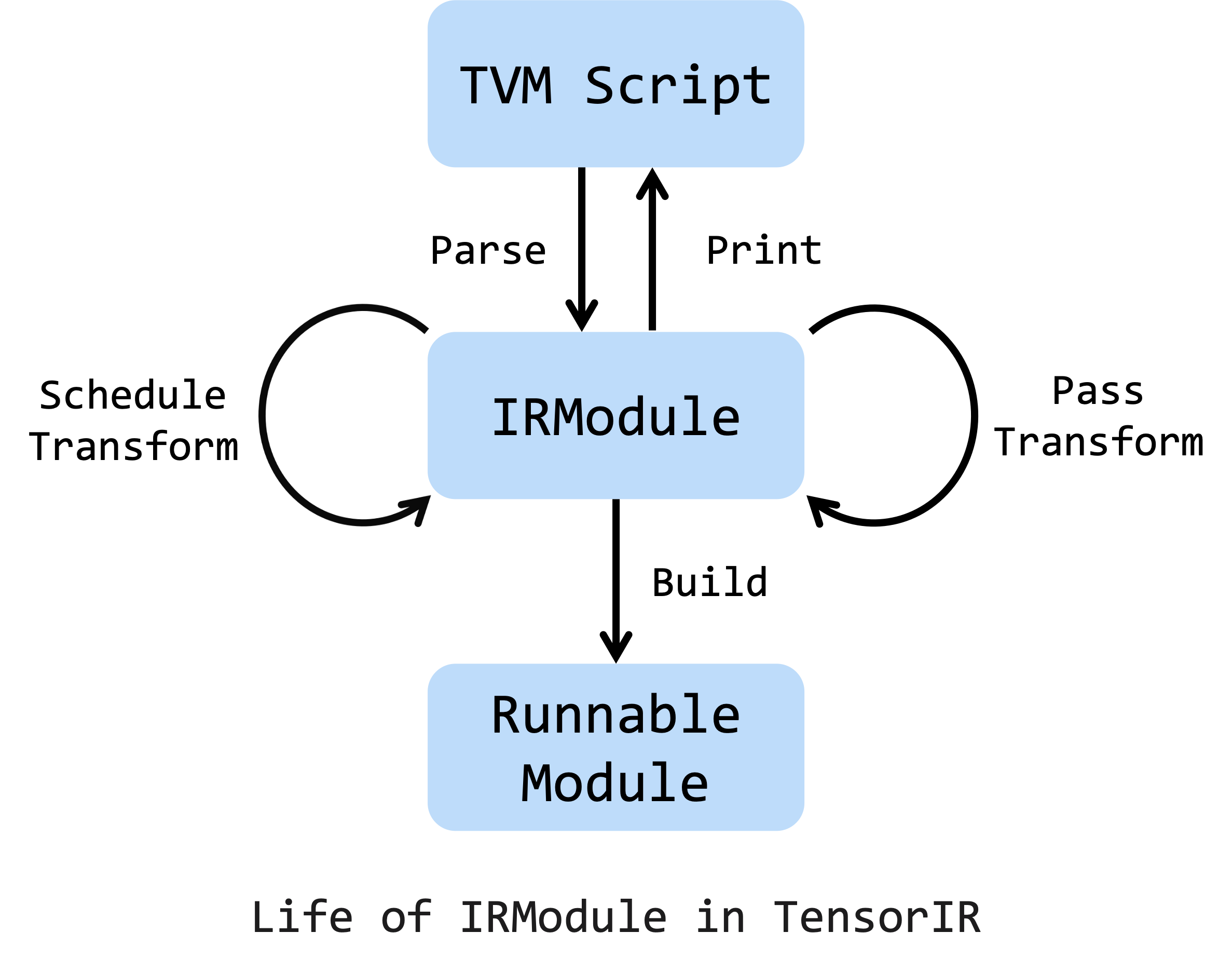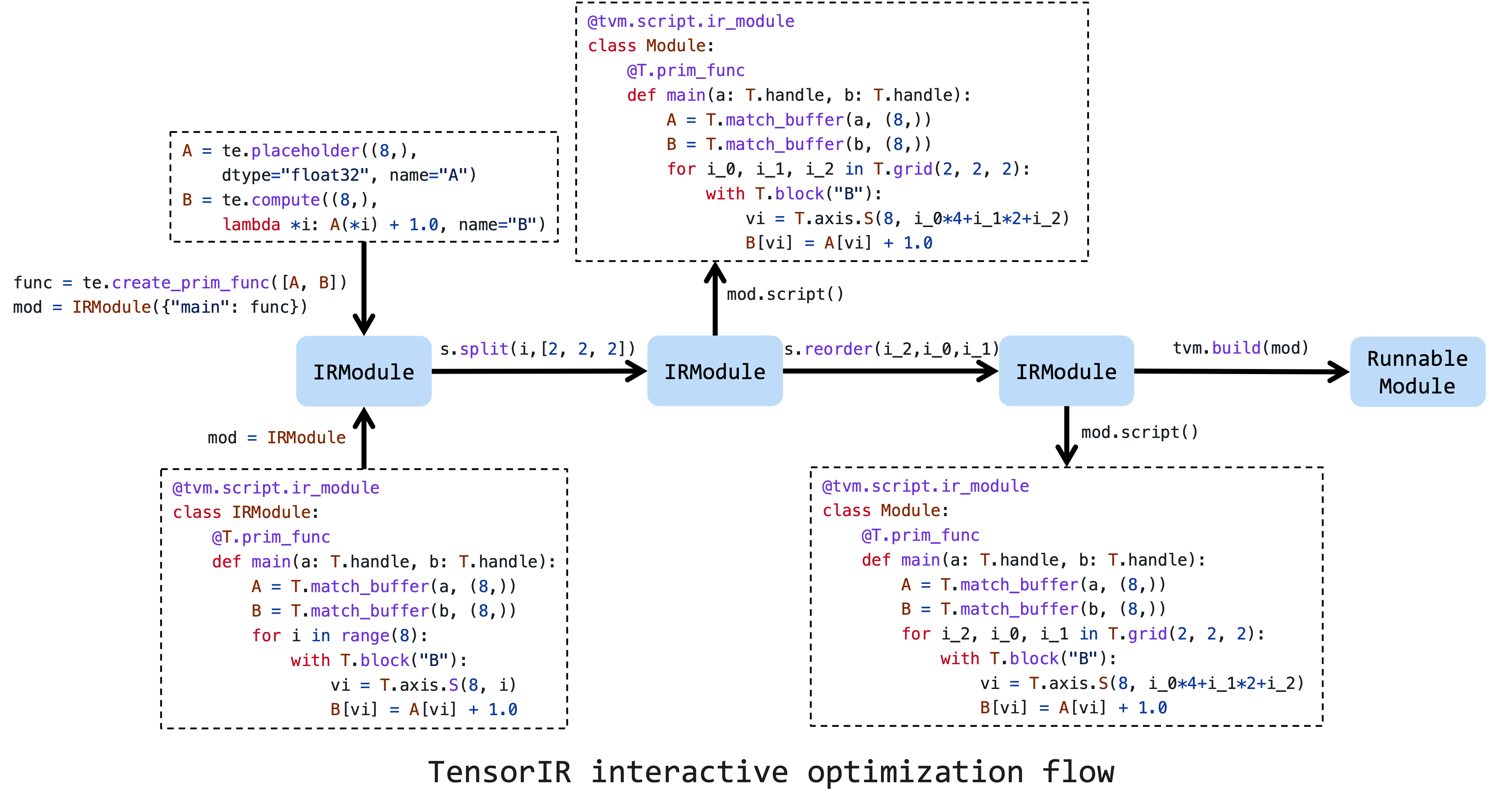# Blitz Course to TensorIR¶

Author: Siyuan Feng

TensorIR is a domain specific language for deep learning programs serving two broad purposes:

• An implementation for transforming and optimizing programs on various hardware backends.

• An abstraction for automatic _tensorized_ program optimization.

```import tvm
from tvm.ir.module import IRModule
from tvm.script import tir as T
import numpy as np
```

## IRModule¶

An IRModule is the central data structure in TVM, which contains deep learning programs. It is the basic object of interest of IR transformation and model building.This is the life cycle of an IRModule, which can be created from TVMScript. TensorIR schedule primitives and passes are two major ways to transform an IRModule. Also, a sequence of transformations on an IRModule is acceptable. Note that we can print an IRModule at ANY stage to TVMScript. After all transformations and optimizations are complete, we can build the IRModule to a runnable module to deploy on target devices.

Based on the design of TensorIR and IRModule, we are able to create a new programming method:

1. Write a program by TVMScript in a python-AST based syntax.

2. Transform and optimize a program with python api.

3. Interactively inspect and try the performance with an imperative style transformation API.

## Create an IRModule¶

IRModule can be created by writing TVMScript, which is a round-trippable syntax for TVM IR.

Different than creating a computational expression by Tensor Expression (Working with Operators Using Tensor Expression), TensorIR allow users to program through TVMScript, a language embedded in python AST. The new method makes it possible to write complex programs and further schedule and optimize it.

Following is a simple example for vector addition.

```@tvm.script.ir_module
class MyModule:
@T.prim_func
def main(a: T.handle, b: T.handle):
# We exchange data between function by handles, which are similar to pointer.
T.func_attr({"global_symbol": "main", "tir.noalias": True})
# Create buffer from handles.
A = T.match_buffer(a, (8,), dtype="float32")
B = T.match_buffer(b, (8,), dtype="float32")
for i in range(8):
# A block is an abstraction for computation.
with T.block("B"):
# Define a spatial block iterator and bind it to value i.
vi = T.axis.spatial(8, i)
B[vi] = A[vi] + 1.0

ir_module = MyModule
print(type(ir_module))
print(ir_module.script())
```
```<class 'tvm.ir.module.IRModule'>
# from tvm.script import ir as I
# from tvm.script import tir as T

@I.ir_module
class Module:
@T.prim_func
def main(A: T.Buffer((8,), "float32"), B: T.Buffer((8,), "float32")):
T.func_attr({"tir.noalias": T.bool(True)})
# with T.block("root"):
for i in range(8):
with T.block("B"):
vi = T.axis.spatial(8, i)
T.writes(B[vi])
B[vi] = A[vi] + T.float32(1)
```

Besides, we can also use tensor expression DSL to write simple operators, and convert them to an IRModule.

```from tvm import te

A = te.placeholder((8,), dtype="float32", name="A")
B = te.compute((8,), lambda *i: A(*i) + 1.0, name="B")
func = te.create_prim_func([A, B])
ir_module_from_te = IRModule({"main": func})
print(ir_module_from_te.script())
```
```# from tvm.script import ir as I
# from tvm.script import tir as T

@I.ir_module
class Module:
@T.prim_func
def main(A: T.Buffer((8,), "float32"), B: T.Buffer((8,), "float32")):
T.func_attr({"tir.noalias": T.bool(True)})
# with T.block("root"):
for i0 in range(8):
with T.block("B"):
v_i0 = T.axis.spatial(8, i0)
T.writes(B[v_i0])
B[v_i0] = A[v_i0] + T.float32(1)
```

## Build and Run an IRModule¶

We can build the IRModule into a runnable module with specific target backends.

```mod = tvm.build(ir_module, target="llvm")  # The module for CPU backends.
print(type(mod))
```
```<class 'tvm.driver.build_module.OperatorModule'>
```

Prepare the input array and output array, then run the module.

```a = tvm.nd.array(np.arange(8).astype("float32"))
b = tvm.nd.array(np.zeros((8,)).astype("float32"))
mod(a, b)
print(a)
print(b)
```
```[0. 1. 2. 3. 4. 5. 6. 7.]
[1. 2. 3. 4. 5. 6. 7. 8.]
```

## Transform an IRModule¶

The IRModule is the central data structure for program optimization, which can be transformed by `Schedule`. A schedule contains multiple primitive methods to interactively transform the program. Each primitive transforms the program in certain ways to bring additional performance optimizations.The image above is a typical workflow for optimizing a tensor program. First, we need to create a schedule on the initial IRModule created from either TVMScript or Tensor Expression. Then, a sequence of schedule primitives will help to improve the performance. And at last, we can lower and build it into a runnable module.

Here we just demonstrate a very simple transformation. First we create schedule on the input ir_module.

```sch = tvm.tir.Schedule(ir_module)
print(type(sch))
```
```<class 'tvm.tir.schedule.schedule.Schedule'>
```

Tile the loop into 3 loops and print the result.

```# Get block by its name
block_b = sch.get_block("B")
# Get loops surrounding the block
(i,) = sch.get_loops(block_b)
# Tile the loop nesting.
i_0, i_1, i_2 = sch.split(i, factors=[2, 2, 2])
print(sch.mod.script())
```
```# from tvm.script import ir as I
# from tvm.script import tir as T

@I.ir_module
class Module:
@T.prim_func
def main(A: T.Buffer((8,), "float32"), B: T.Buffer((8,), "float32")):
T.func_attr({"tir.noalias": T.bool(True)})
# with T.block("root"):
for i_0, i_1, i_2 in T.grid(2, 2, 2):
with T.block("B"):
vi = T.axis.spatial(8, i_0 * 4 + i_1 * 2 + i_2)
T.writes(B[vi])
B[vi] = A[vi] + T.float32(1)
```

We can also reorder the loops. Now we move loop i_2 to outside of i_1.

```sch.reorder(i_0, i_2, i_1)
print(sch.mod.script())
```
```# from tvm.script import ir as I
# from tvm.script import tir as T

@I.ir_module
class Module:
@T.prim_func
def main(A: T.Buffer((8,), "float32"), B: T.Buffer((8,), "float32")):
T.func_attr({"tir.noalias": T.bool(True)})
# with T.block("root"):
for i_0, i_2, i_1 in T.grid(2, 2, 2):
with T.block("B"):
vi = T.axis.spatial(8, i_0 * 4 + i_1 * 2 + i_2)
T.writes(B[vi])
B[vi] = A[vi] + T.float32(1)
```

### Transform to a GPU program¶

If we want to deploy models on GPUs, thread binding is necessary. Fortunately, we can also use primitives and do incrementally transformation.

```sch.bind(i_0, "blockIdx.x")
print(sch.mod.script())
```
```# from tvm.script import ir as I
# from tvm.script import tir as T

@I.ir_module
class Module:
@T.prim_func
def main(A: T.Buffer((8,), "float32"), B: T.Buffer((8,), "float32")):
T.func_attr({"tir.noalias": T.bool(True)})
# with T.block("root"):
for i_1 in range(2):
with T.block("B"):
vi = T.axis.spatial(8, i_0 * 4 + i_1 * 2 + i_2)
T.writes(B[vi])
B[vi] = A[vi] + T.float32(1)
```

After binding the threads, now build the IRModule with `cuda` backends.

```ctx = tvm.cuda(0)
cuda_mod = tvm.build(sch.mod, target="cuda")
cuda_a = tvm.nd.array(np.arange(8).astype("float32"), ctx)
cuda_b = tvm.nd.array(np.zeros((8,)).astype("float32"), ctx)
cuda_mod(cuda_a, cuda_b)
print(cuda_a)
print(cuda_b)
```
```[0. 1. 2. 3. 4. 5. 6. 7.]
[1. 2. 3. 4. 5. 6. 7. 8.]
```

Gallery generated by Sphinx-Gallery